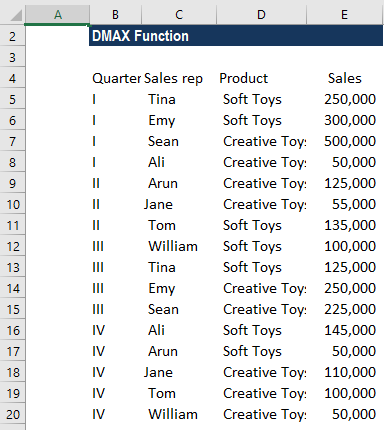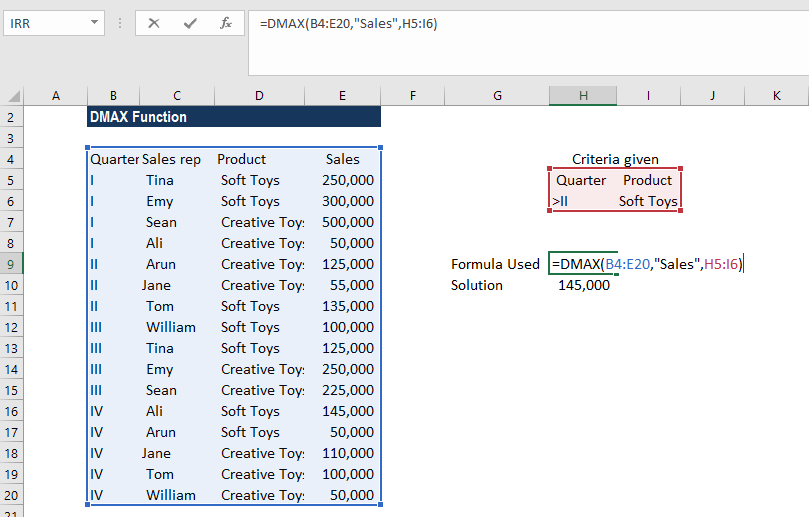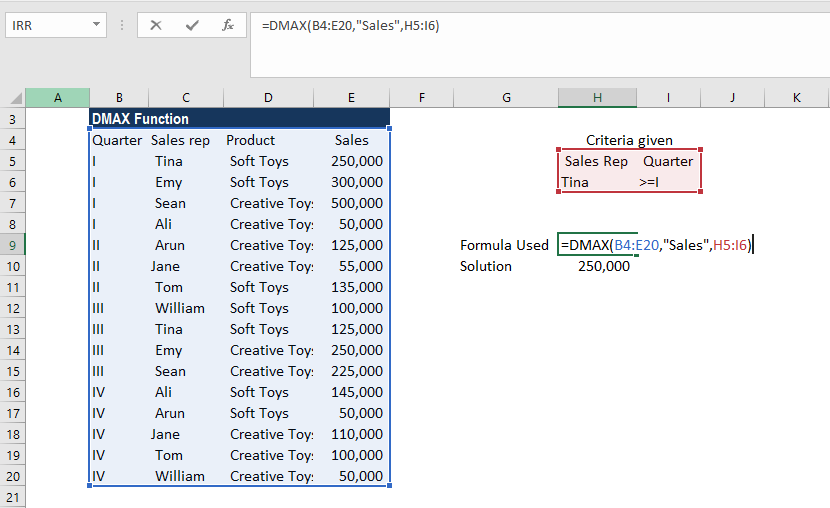# DMAX Function

Returns the maximum value for a specific field/column in a database

## What is the DMAX Function?

The DMAX Function is categorized under Excel Database functions. The function helps find the maximum value for a specific field/column in a database for selected records based on user-specified criteria.

DMAX will return a numerical value. It was introduced in MS Excel 2000.

### Formula

=DMAX(database, field, criteria)

The DMAX function uses the following arguments:

1. Database (required argument) – Database is the range of cells wherein the first row of the database specifies the field names.
2. Field (required argument) – This is the column within the database from which we wish to find the maximum value. Field can be a field name, that is the header provided on the top row such as “Sales rep,” “Sales,” etc., or it can be a number.
3. Criteria (required argument) – This is a range of cells that contains the criteria specified. It specifies the record that will be included in the calculation. We can use any range for the criteria argument as long as it includes at least one column label and at least one cell below the column label in which the condition for the column is specified.

The criteria argument provided can be either:

• A numeric value (including an integer, decimal, date, time, or logical value) (e.g. 10, 10/01/2017, FALSE)
• A text string (e.g. “North”, “Friday”)
• An expression (e.g. “>15”, “<>0”).

### How to use the DMAX Function in Excel?

To understand the uses of the function, let’s consider a few examples:

#### Example 1

Suppose we are given the data below:If we wish to find the highest sales figure by any sales rep during quarters 3 and 4, we can use the DMAX function. In the example, the criteria are specified in cells H5-I6 and the DMAX formula is shown below:The highest sales of soft toys were \$145,000, which was made by Ali. In case there are more than two sales reps, one way would be to use a data filter and find out the reps who achieved the maximum sales.

#### Example 2

Continuing with the same data, suppose we need data that tells us the highest sales made by Tina. The criteria specified are:

• Sales Rep: Tina
• Quarter: >=1

We need to put ‘>=’ as we want the highest sales from all four quarters.

The formula used is:The highest sales made by Tina are \$250,000.

### Things to remember about the DMAX Function:

1. The criteria range can be located anywhere on the worksheet, but it is advisable to not place the criteria range below the list. If we add more information to the list, the new information is added to the first row below the list. If the row below the list is not blank, MS Excel cannot add the new information.
2. We should make sure that the criteria range does not overlap the list.
3. If we wish to perform an operation on an entire column in a database, we need to enter a blank line below the column labels in the criteria range.
4. Remember that the Excel database functions allow wildcards to be used in text-related criteria such as ? (matches any single character) and * (matches any sequence of characters).

However, if we wish to find out a “?” or “*” then we will need to type the ~ (tilde) symbol before the character in your search. For example, the condition “m*e” will match all cells containing a text string beginning with “m” and ending in “e.”

Thanks for reading CFI’s guide to important Excel functions! By taking the time to learn and master these Excel functions, you’ll significantly speed up your financial analysis. To learn more, check out these additional CFI resources:

• Excel Functions for Finance
• Advanced Excel Formulas Course
• Advanced Excel Formulas You Must Know
• Excel Shortcuts for PC and Mac

### Free Excel Tutorial

To master the art of Excel, check out CFI’s FREE Excel Crash Course, which teaches you how to become an Excel power user.  Learn the most important formulas, functions, and shortcuts to become confident in your financial analysis.

Launch CFI’s Free Excel Course now to take your career to the next level and move up the ladder!